## Figures index

#### V.S. Olkhovsky

American Journal of Applied Mathematics and Statistics. 2015, 3(3), 111-130 doi:10.12691/ajams-3-3-5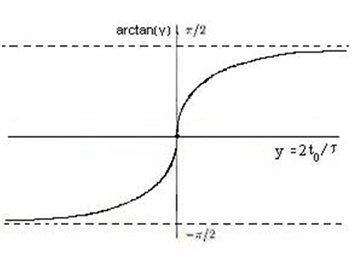• Figure 1. The function arctan(y) for 2t0n→∞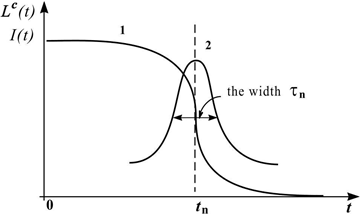• Figure 2. Lc(τ) (the curve 1) and I(t) (the curve 2)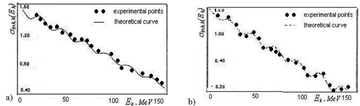• Figure 3. The inclusive process p + C 7Be +X (protons of 2.1 GeV), experimental data are taken from : a) C1= 0.04, C2= 0.36 (θ = 90); b) C1= 0.35, C2= 0.05 (θ = 160)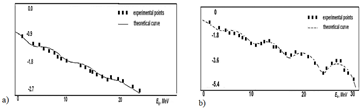• Figure 4. The inclusive process 4He + Ta t+ X (720 MeV/nucleon), experimental data are taken from : a) C1=0.18, C2=1.02 (θ=60o); b) C1=1.13, C2=0.07 (θ=90o)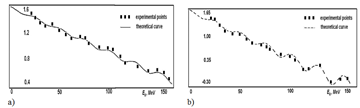• Figure 5. The inclusive process 20Ne +U p+ X (1045 MeV/nucleon), experimental data are taken from : a) C1=0.35, C2=5.65 (θ=90o); b) C1=5.65, C2=0.35 (θ=150o)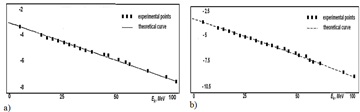• Figure 6. The inclusive process 40Ar + 51V p+ X (41 MeV/nucleon); experimental data are taken from 36: а) C1=0.002, C2=0.03 (θ=97o); b) C1=0.03, C2=0.022 (θ=129o)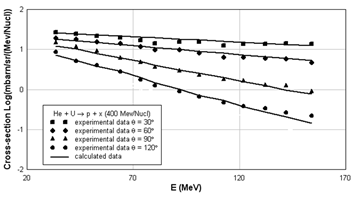• Figure 7. Inclusive energy spectrum of 4He + Up+ X, of 400 MeV/nucleon, experimental data are taken from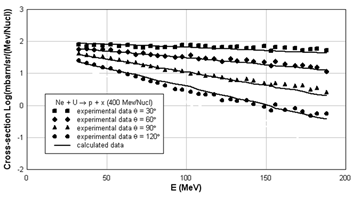• Figure 8. Inclusive energy spectrum of 20Ne+ U p+ X, of 400 MeV/nucleon, experimental data are taken from